# 绘图功能

### 如何使用

• 小数必须由点分离
•
• 您可以不写乘号
• 在某些情况下，
• 您可以在同一时间建立了一套功能图表
•
• 您可以自定义轴的名称和它们的间隔
•
• 时间表可以被下载为PNG图像
•
• 可以打印该图
•
• 您可以得到的时间表参照与他人分享
•
• 当光标在图形上，它可以移动和放大或缩小
•

### 模式

• 普通 — 在这种模式下，你可以建立由以下公式定义的图形功能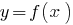• 参数 — 可以绘制参数不限定的曲线，即，以的形式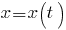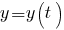• 极坐标 — 可以绘制极坐标系中定义的曲线，即，方程, 哪里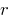— 径向坐标, 和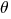— 极坐标
• 在点 — 这种模式的目的是为了表明他们的点的坐标函数的图形建设

### 运营商

`+` 加成
`-` 减法
`*` 乘法
`/`
`^n` 取幂n
`^(1/n)` 第n度的根
`()` 括号

### 功能

`abs(x)` 模块x
`sqrt(x)` x的平方根
`nthRoot(x,n)` x的第n度的根
`pow(x,n)` 建设x到电力n
`exp(x)` x的指数（欧拉的幂数x）
`log2(x)` x的基数2对数
`log10(x)` x的基数10对数
`ln(x)` x的自然对数
`min(...)` 数字的最小值
`max(...)` 最大值从一组数字

### 三角函数

`sin(x)` x的正弦
`cos(x)` x的余弦
`tan(x)` x的切线
`1/tan(x)` 少许的x
`asin(x)` x的反正弦
`acos(x)` x的余弦余弦
`atan(x)` x的反正切
`PI_HALF-atan(x)` 弧的余光x
`sinh(x)` x的双曲正弦
`cosh(x)` x的双曲余弦
`tanh(x)` x的双曲正切
`1/tanh(x)` x的双曲余。

### 常量

`PI` 数字Pi（3.14159 ...）
`PI_HALF` 数Pi除以2
`PI_TWICE` 数字Pi乘以2
`ONE`
`ZERO`